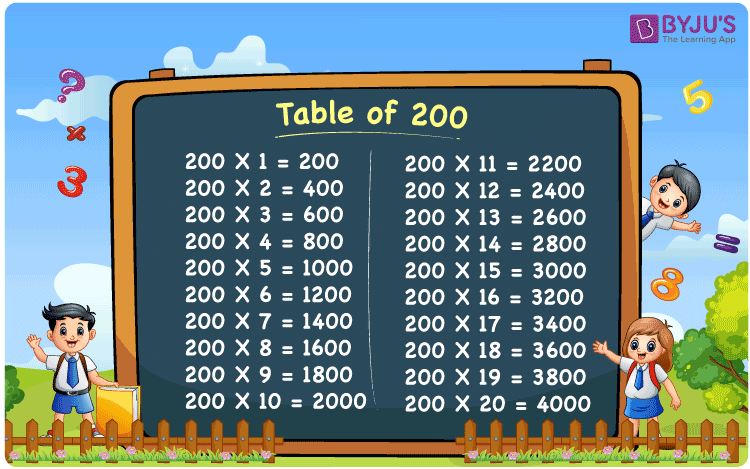Checkout JEE MAINS 2022 Question Paper Analysis : Checkout JEE MAINS 2022 Question Paper Analysis :

# Table of 200

Table of 200 is the multiplication table that expresses the product values of 200 multiplied by consecutive natural numbers. The values in the 200 times table can be produced by repeated addition of the original number. For example, 200 times 3, 200 x 3 = 200 + 200 + 200 = 600. The table of 200 is easy to remember if you know the table of 2. You just need to multiply the multiples of 2 with 100. Like 2 times 4 is 8 and 8 multiplied by 100 is 800, which is equal to 200 multiplied by 4. Learn more about multiplication tables at BYJU’S. You can also download the PDF of table 200 for free here.

## Table of 200 Chart## What is the 200 Times Table?

Learn how to write the 200 times table, with the help of the repeated addition table given below.

 200×1 = 200 200 200×2 = 400 200 + 200 = 400 200×3 = 600 200 + 200 + 200 = 600 200×4 = 800 200 + 200 + 200 + 200 = 800 200×5 = 1000 200 + 200 + 200 + 200 + 200 = 1000 200×6 = 1200 200 + 200 + 200 + 200 + 200 + 200 = 1200 200×7 = 1400 200 + 200 + 200 + 200 + 200 + 200 + 200 = 1400 200×8 = 1600 200 + 200 + 200 + 200 + 200 + 200 + 200 + 200 = 1600 200×9 = 1800 200 + 200 + 200 + 200 + 200 + 200 + 200 + 200 + 200 = 1800 200×10 = 2000 200 + 200 + 200 + 200 + 200 + 200 + 200 + 200 + 200 + 200 = 2000

## Multiplication table of 200

Here is the table of 200 up to 20 times below.

 200 × 1 = 200 200 × 2 = 400 200 × 3 = 600 200 × 4 = 800 200 × 5 = 1000 200 × 6 = 1200 200 × 7 = 1400 200 × 8 = 1600 200 × 9 = 1800 200 × 10 = 2000 200 × 11 = 2200 200 × 12 = 2400 200 × 13 = 2600 200 × 14 = 2800 200 × 15 = 3000 200 × 16 = 3200 200 × 17 = 3400 200 × 18 = 3600 200 × 19 = 3800 200 × 20 = 4000

## Solved Examples on Table of 200

Q.1: What is the value of 200 multiplied by 5 plus 50? Write the answer in words.

Solution: 200 multiplied by 5 = 200 x 5 = 1000

Hence,

200 x 5 + 50

1000 + 50

1050

Therefore, the answer is One Thousand and Fifty.

Q.2: The cost of a pair of slippers is Rs. 200. What is the cost of 4 such pairs of slippers?

Solution: Given,

Cost of a pair of slippers = Rs.200

Cost of 4 pairs of slippers = Rs. 200 x 4 = Rs. 800

## Frequently Asked Questions on Table of 200

### What is table of 200?

Table of 200 is the multiplication table that represents the product of 200 by a list of consecutive natural numbers.

### What is 200 times 6 equal to?

From the table of 200, 200 times 6 is equal to 1200.

### How many times 200 should be added to itself to get 1400?

200 times should be added to seven times to get 1400. 200 x 7 = 1400.

### What should be multiplied by 200 to get 2800?

From the table of 200, we need to multiply 200 by 14, which is equal to 2800.